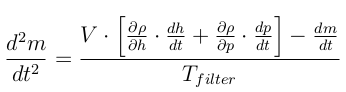# Filtering Method

This method is applied at the Modelica level to deal with the discontinuous density derivative between liquid and two-phase in finite volume models.

In this strategy , a first order filter is applied to the fast variations of the density with respect to time This filter therefore acts as ”mass damper” and avoids transmitting abrupt variations of the flow rate due the density derivative discontinuity. The filtered mass accumulation in each cell is written:where Tfilter is the filter time constant, set as model input. This strategy displaces the mass variations in time but does not generate mass defects. However the energy balance is affected because the cell density is not exactly the one corresponding to the actual node flow rates. The error of this method on the simulation results and on the heat balances can be significant. It should therefore be used with care.

This approach can be implemented at the model level, but doubles the number of time states of the model since a second-order derivative of the working fluid mass in the cell is defined.

# Reference

Sylvain Quoilin, Ian Bell, Adriano Desideri, and Vincent Lemort. Methods to increase the robustness of finite-volume flow models in thermodynamic systems. To be published in Energies, 2014.